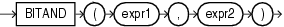# BITAND

SyntaxDescription of the illustration bitand.gif

Purpose

The `BITAND` function treats its inputs and its output as vectors of bits; the output is the bitwise `AND` of the inputs.

The types of `expr1` and `expr2` are `NUMBER`, and the result is of type `NUMBER`. If either argument to `BITAND` is `NULL`, the result is `NULL`.

The arguments must be in the range -(2(n-1)) .. ((2(n-1))-1). If an argument is out of this range, the result is undefined.

The result is computed in several steps. First, each argument A is replaced with the value `SIGN(A)*FLOOR(ABS(A))`. This conversion has the effect of truncating each argument towards zero. Next, each argument A (which must now be an integer value) is converted to an n-bit two's complement binary integer value. The two bit values are combined using a bitwise `AND` operation. Finally, the resulting n-bit two's complement value is converted back to `NUMBER`.

Notes on the BITAND Function

• The current implementation of `BITAND` defines `n` = 128.

• PL/SQL supports an overload of `BITAND` for which the types of the inputs and of the result are all `BINARY_INTEGER` and for which `n` = 32.

Examples

The following example performs an `AND` operation on the numbers 6 (binary 1,1,0) and 3 (binary 0,1,1):

```SELECT BITAND(6,3) FROM DUAL;

BITAND(6,3)
-----------
2
```

This is the same as the following example, which shows the binary values of 6 and 3. The `BITAND` function operates only on the significant digits of the binary values:

```SELECT BITAND(
BIN_TO_NUM(1,1,0),
BIN_TO_NUM(0,1,1)) "Binary"
FROM DUAL;

Binary
----------
2
```

Refer to the example for BIN_TO_NUM for information on encoding multiple values in a single column value.

The following example supposes that the `order_status` column of the sample table `oe.orders` encodes several choices as individual bits within a single numeric value. For example, an order still in the warehouse is represented by a binary value 001 (decimal 1). An order being sent by ground transportation is represented by a binary value 010 (decimal 2). An insured package is represented by a binary value 100 (decimal 4). The example uses the `DECODE` function to provide two values for each of the three bits in the `order_status` value, one value if the bit is turned on and one if it is turned off.

```SELECT order_id, customer_id, order_status,
DECODE(BITAND(order_status, 1), 1, 'Warehouse', 'PostOffice')
"Location",
DECODE(BITAND(order_status, 2), 2, 'Ground', 'Air') "Method",
DECODE(BITAND(order_status, 4), 4, 'Insured', 'Certified') "Receipt"
FROM orders
WHERE sales_rep_id = 160
ORDER BY order_id;

ORDER_ID CUSTOMER_ID ORDER_STATUS Location   Method Receipt
---------- ----------- ------------ ---------- ------ ---------
2455         145            7 Warehouse  Ground Insured
2416         104            6 PostOffice Ground Insured
2419         107            3 Warehouse  Ground Certified
2420         108            2 PostOffice Ground Certified
2423         145            3 Warehouse  Ground Certified
2441         106            5 Warehouse  Air    Insured
```

For the `Location` column, `BITAND` first compares `order_status` with 1 (binary 001). Only significant bit values are compared, so any binary value with a 1 in its rightmost bit (any odd number) will evaluate positively and return 1. Even numbers will return 0. The `DECODE` function compares the value returned by `BITAND` with 1. If they are both 1, then the location is "Warehouse". If they are different, then the location is "PostOffice".

The `Method` and `Receipt` columns are calculated similarly. For `Method`, `BITAND` performs the `AND` operation on `order_status` and 2 (binary 010). For `Receipt`, `BITAND` performs the `AND` operation on `order_status` and 4 (binary 100).